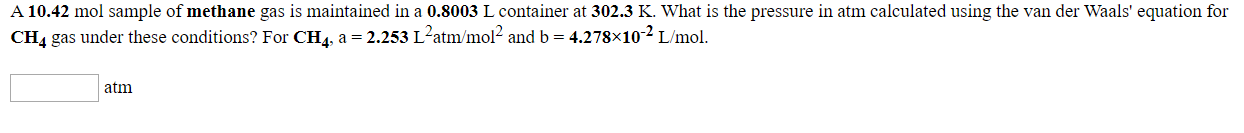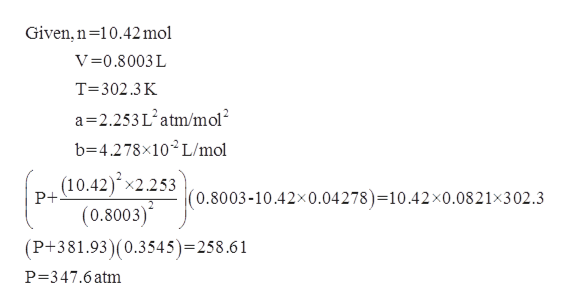# A 10.42 mol sample of methane gas is maintained in a 0.8003 L container at 302.3 K. What is the pressure in atm calculated using the van der Waals' equation fora 2.253 L2atm/mol2 and b = 4.278x102 L/mol.CH4 gas under these conditions? For CH4atm

Question
15 viewshelp_outlineImage TranscriptioncloseA 10.42 mol sample of methane gas is maintained in a 0.8003 L container at 302.3 K. What is the pressure in atm calculated using the van der Waals' equation for a 2.253 L2atm/mol2 and b = 4.278x102 L/mol. CH4 gas under these conditions? For CH4 atm fullscreen
check_circle

Step 1

The vander Waals’ equation is given as

Step 2

For calculating the pressure of methane gas, the gi...help_outlineImage TranscriptioncloseGiven, n 10.42 mol V=0.8003 L T 302.3 K a 2.253L atm/mol b 4.278x10 L/mol (10.42)x2.253 P+ |(0.8003-10.42x0.04278)=10.42 x0.0821x302.3 (0.8003) (P+381.93)(0.3545)=258 .6 1 P 347.6atm fullscreen

### Want to see the full answer?

See Solution

#### Want to see this answer and more?

Solutions are written by subject experts who are available 24/7. Questions are typically answered within 1 hour.*

See Solution
*Response times may vary by subject and question.
Tagged in

### Physical Chemistry